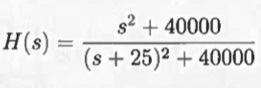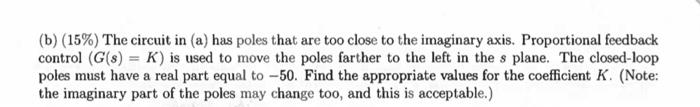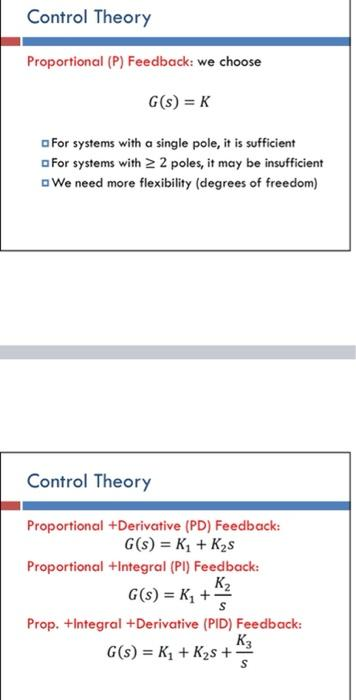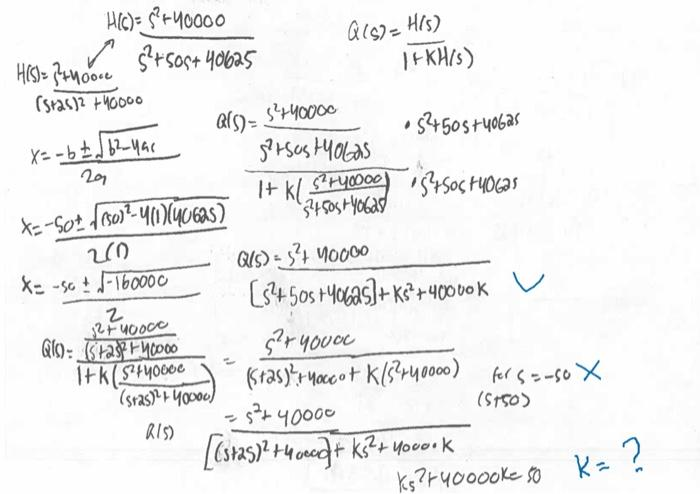# (Solved): the transfert function is attached. he was close to find the answers but apparently did something wr ...

the transfert function is attached. he was close to find the answers but apparently did something wrong along the way(b) (15\%) The circuit in (a) has poles that are too close to the imaginary axis. Proportional feedback control is used to move the poles farther to the left in the plane. The closed-loop poles must have a real part equal to -50 . Find the appropriate values for the coefficient . (Note: the imaginary part of the poles may change too, and this is acceptable.) Proportional (P) Feedback: we choose For systems with a single pole, it is sufficient afor systems with poles, it may be insufficient We need more flexibility (degrees of freedom) Control Theory Proportional +Derivative (PD) Feedback: Proportional +Integral (PI) Feedback: Prop. + Integral +Derivative (PID) Feedback:

We have an Answer from Expert